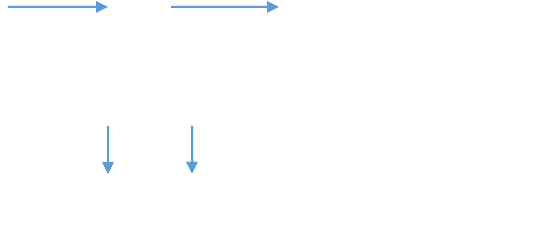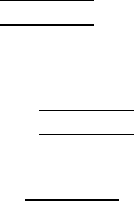Study Guides (400,000)
CA (150,000)
UTSG (10,000)
MAT (1,000)
MAT135H1 (100)
all (40)
Quiz

# MAT135H1 Study Guide - Quiz Guide: Quadratic Function, Product Rule, Graph Of A Function

Department
Mathematics
Course Code
MAT135H1
Professor
all
Study Guide
Quiz

This preview shows pages 1-3. to view the full 26 pages of the document.

I
Fall 2015
Term Test 1
Exam Guide
www.OneClass.com
www.OneClass.com
www.OneClass.com
www.OneClass.com

Only pages 1-3 are available for preview. Some parts have been intentionally blurred.Dependent
Variable
Independent
Variable
Calculus I Early Transcendentals ( 8 Edition )
by James Stewart
Chapter 1 Functions and Models
1.1 Four Ways to Represent a Function
1. What’s Function?
A function f is a rule that assigns to each elements x in a set D exactly one element, called f(x), in set E.
x f f(x)
Example: 
A is a function of r, the number of A is called the value of A at r. If r = 2, the A = 4π. 4π is the value of A at
r = 2.
Important Note:
Composed Functions:
Given two functions f and g, the composed function f ◦ g is defined by: (f ◦ g) = f (g ()).
Past Exam Question:
1. f() = +1, g() = . Find f ◦ g and g ◦ f.
Solution:
f ◦ g() = f (g ()) g ◦ f(x) = g (f())
= f () = g ( +1)
= = 
2. f() = +2, g() = , h() = 2. Find f ◦ g ◦ h.
Solution:
f ◦ g ◦ h () = f ( g ( h() ) )
= f ( g (2 ) )
= f ()
www.OneClass.com
www.OneClass.com
www.OneClass.com
www.OneClass.com

Only pages 1-3 are available for preview. Some parts have been intentionally blurred.= f ( 4) = ( 4) +2 = 4 + 2
2. Domain & Range
Domain: The domain of a function denoted by f is the set of points f is defined on.
Range: The range of a function denoted by f is the set of values that f(x) takes.
Example:
f() = , x R, f(x) .
Domain: (
Range: [ 0, 
Important Note:
Let f(x) domain is D, g(x) domain is E, then, the domain of f ◦ g() = f (g ()) is ;
Similarly, the domain of g ◦ f(x) = g (f()) is .
Past Exam Question:
1. f(x) = 2x + 3, for all x  g(x) = x 1, , for all x . Find f ◦ g() and state its domain.
Solution:
f ◦ g() = f (g ()) = f ( x 1) = 2(x 1) + 3
= 2x + 1
The domain of f ◦ g() = 
= 
= 
= 
= 
2. Find the domain of f(x) =
.
Solution:
The domain of f(x) = 

= 

www.OneClass.com
www.OneClass.com
www.OneClass.com
www.OneClass.com